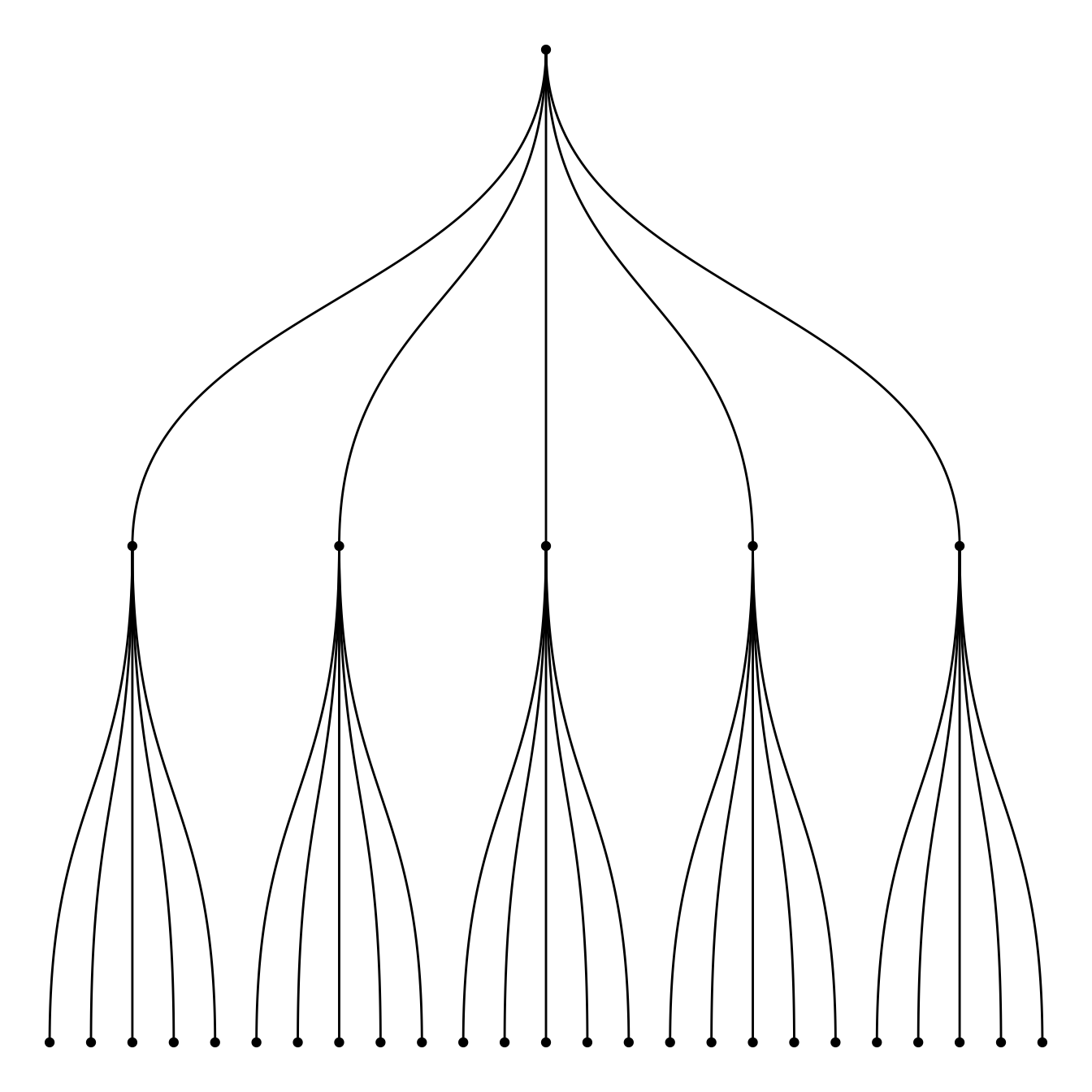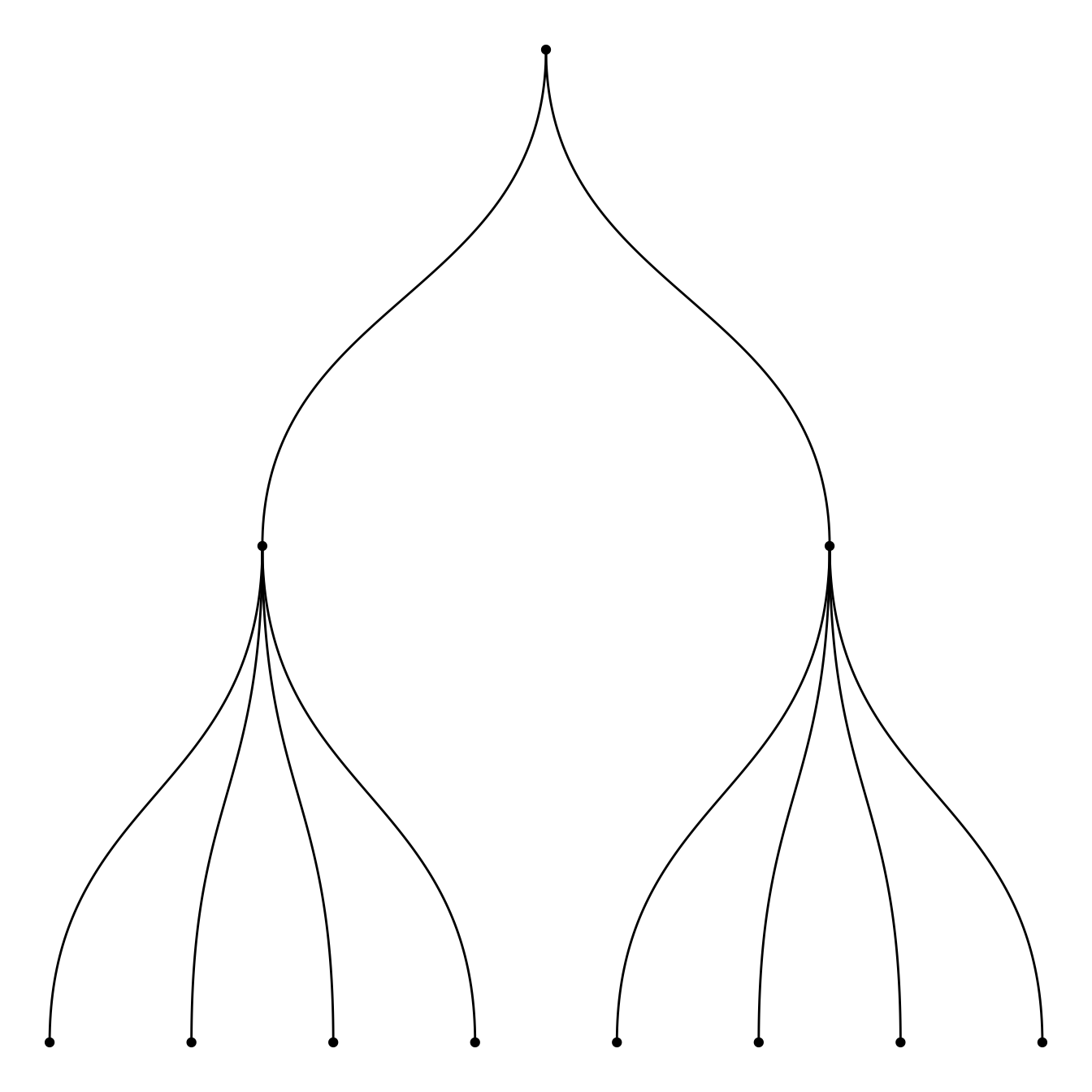# Introduction to tree diagram with R and ggraph

This posts is a step by step introduction to building dendrograms with R and the `ggraph` package. It provides several examples based on different input formats with explanation and reproducible code.

This page aims to describe how to make a basic dendrogram representing hierarchical data with the `ggraph` library. Two input formats are considered:

• edge list → 2 columns, one row is on connection
• nested data frame → one row is one path from root to leaf. As many columns as the number of levels in the hierarchy

Please visit this page to learn how to custom these dendrograms. If you want to create a dendrogram from clustering result, visit the dendrogram section of the gallery.

# Dendrogram from edge list

Edge list is the most convenient format to use `ggraph`. Follow those steps:

• transform the input dataframe to a graph object using the `graph_from_data_frame()` function from the `igraph` library
• use the dendrogram layout of `ggraph` with `layout = 'dendrogram'`Edge list data format``````# libraries
library(ggraph)
library(igraph)
library(tidyverse)

# create an edge list data frame giving the hierarchical structure of your individuals
d1 <- data.frame(from="origin", to=paste("group", seq(1,5), sep=""))
d2 <- data.frame(from=rep(d1\$to, each=5), to=paste("subgroup", seq(1,25), sep="_"))
edges <- rbind(d1, d2)

# Create a graph object
mygraph <- graph_from_data_frame( edges )

# Basic tree
ggraph(mygraph, layout = 'dendrogram', circular = FALSE) +
geom_edge_diagonal() +
geom_node_point() +
theme_void()``````

# Dendrogram from a nested dataframe

Another common format is the nested data frame. The code below shows how to easily transform it into a nested data frame. Once it is done, just apply the code described above once more.Nested dataframe format``````# libraries
library(ggraph)
library(igraph)
library(tidyverse)

# create a data frame
data <- data.frame(
level1="CEO",
level2=c( rep("boss1",4), rep("boss2",4)),
level3=paste0("mister_", letters[1:8])
)

# transform it to a edge list!
edges_level1_2 <- data %>% select(level1, level2) %>% unique %>% rename(from=level1, to=level2)
edges_level2_3 <- data %>% select(level2, level3) %>% unique %>% rename(from=level2, to=level3)
edge_list=rbind(edges_level1_2, edges_level2_3)

# Now we can plot that
mygraph <- graph_from_data_frame( edge_list )
ggraph(mygraph, layout = 'dendrogram', circular = FALSE) +
geom_edge_diagonal() +
geom_node_point() +
theme_void()``````

Related chart types

## Contact

This document is a work by Yan Holtz. Any feedback is highly encouraged. You can fill an issue on Github, drop me a message on Twitter, or send an email pasting yan.holtz.data with gmail.com.